## Monday, November 24, 2014

### Inertial and Space Warp Principles

Translation of Angular Momentum Into Directional Motion:
Davis Mechanics and Loop Holes in the Third Law of Motion

Edward M. Renner
Original: 4/1985; Revised: 9/2011, 10/2013, & 1/2014

Introduction

There are many grey areas in classical Newtonian physics that cannot explain anomalous mechanical behavior or transient phenomena. Yet, from investigation of some of these anomalies have come innovative ideas and new concepts which have expanded the scope of classical physics to include relativistic physics… and the concept of inertial drives. The concept of an inertial drive has been around for many years, from science fiction to dozens of interesting inventions and devices. Most of these inventions usually depend on known scientific phenomena to manifest their apparent violations of the laws` of motion (i.e., slip-stick friction, precession). However, when anomalous phenomena (deviations from theory) consistently occur under specific and controlled experimental conditions, it is probably the mathematical model that is at fault or incomplete, not the results; thus experimentation with inertial effects and other discoveries in the engineering field (when the existing paradigms failed to explain experienced phenomena) have also resulted in a rethinking/reformulation of time honored mathematical theories and classical motion laws. Although the concept itself is simple, its reality appears to violate what we accept as classical laws of motion/ momentum (i.e., 3rd Law); thus has generally been ignored, ridiculed, or explained away by most theoretical scientists. However, there are many examples of special case / apparent violations of motion laws from the annals of engineering and science, and there are many simple proof-of-principle devices that can be, and have been, created to demonstrate these apparent violations and isolate the phenomena of interest. The governing principles of real inertial drives were perhaps best summed up mathematically by William O. Davis in his Fourth Law of Motion (1962, Davis Mechanics); a law that accounts for the various transient and anomalous inertial effects seen in real physical systems (see Discussion).

This paper is a review of angular momentum translation principles/ experiments, the transient effects seen in forced harmonic driving forces, and the theoretical foundation of inertial drives; plus speculations on the consequences and applications of such drives.

Principles and Devices

Conservation and Conversion of Momentum
The Conservation of Momentum is a principle that has real applications only at relatively low velocities and under idealized conditions. This is quite apparent when you consider that momentum (mv) is converted in a high velocity impact, via kinetic energy (mv2/2) and friction, into random molecular momentum (heat). Thus the conservation of momentum is a principle with many exceptions in physical reality, and is simply a reflection of the conservation of total energy (SE); i.e.,

ES 1 = [mv1]~ mv12/2 --->[mv2] ~ mv22/2 + ΔH = ES 2 ; mv2 < mv1, but ES 2 =ES 1

Therefore, the conversion of angular momentum into linear momentum (a proven principle) without an intermediate factor (i.e., friction, impact) is not a violation of any physical law if total energy is conserved.

ES = Iw12/2 -à linear conversion ---® Iw2 2 /2 + mv1 2/2 = ES

One way this conversion can be demonstrated is by amplifying certain transient effects found in rotating masses undergoing forced harmonic and precessional motion.

The Drive Wheel
The simplest form of translation of angular momentum (Iw ) to linear momentum (mv) is demonstrated by the ordinary drive wheel; e.g., the wheel of a car. In order for a car, bicycle, or train to move forward, the angular momentum generated by the engine/driving force and imparted to the wheel must be translated via friction and contact with the frictional contact surface (the road, rail, etc.). This happens by a maximum deceleration of the wheel occurring at the interface of wheel-to-surface and a transfer of energy via friction. Maximum acceleration in the wheel occurs at the opposite side of the wheel’s contact with the frictional surface, thus during a rotation cycle of the wheel, one side is at maximum angular velocity while the other side is at a minimum. The net result is that angular momentum is translated into linear momentum/ motion via the intermediary of friction. The actual changes in acceleration and deceleration are real in frictional contact surfaces that are elastic or semi-elastic, but are likely manifest as virtual changes or stored static stress forces when contact surfaces are inelastic, i.e., a steel train wheel. This is a key concept when looking to reassess the conservation of momentum, for in order to have a rigid/inelastic wheel function, there is probably a decoupling of actual and virtual centers-of-mass (or cg from cm).FIGURE 1 - Angular to Linear Momentum in the Drive Wheel via Friction.

The Pendulum/Swing
The pendulum provides an elegant proof of energy conservation and compliance with the basic laws of motion, but it can also be used to demonstrate the effects of a harmonic driving force, translation of one form of energy to another (which the pendulum depends on for its operation), and the amplification and conversion of angular momentum into unidirectional momentum and vice versa.

A functioning pendulum utilizes gravity (g) to transform potential energy (PE) into kinetic energy (KE)/momentum (mv/Iw ) and then back again as it completes one cycle of oscillation (simple harmonic motion). A pendulum bob in motion gradually loses energy due to air resistance and friction; but can be made to oscillate continuously if a small driving force, such as an impulse from an electro-magnet, is used to replenish the energy lost (a Foucault pendulum uses this method to operate). The pendulum can also be made to oscillate from rest by translation of bob internal energy/momentum into mv/Iw via a harmonic driving force; i.e., if the bob of a pendulum is a hollow sphere which contains a smaller mass which oscillates back-and-forth and provides a harmonic driving force at the fundamental frequency of the pendulum, the pendulum will move from rest and oscillate in increasing amplitude (like a child’s swing when the child shifts its’ center of mass to-and-fro at the fundamental frequency of the swing). Also, if an eccentric rotating flywheel is substituted for the linear oscillating mass inside the pendulum bob, the pendulum will oscillate in an elliptical/circular fashion (a conic pendulum) if the rotation rate of the eccentric flywheel is matched to the fundamental frequency of the pendulum. This type of harmonic driving force will produce oscillations of increasing magnitude (limited by the balance between potential and gravitational forces) which essentially is an angular Iw deflection of the “static” acceleration produced by gravity.

Further, if the string that holds the sphere in both of the above systems is cut at a point of maximum kinetic energy/angular momentum, the bob will travel in a tangential (straight) line with equivalent linear momentum (just like a child launching himself off a swing). So it would seem that internal oscillatory momentum may be amplified and stored in a pendulum/ swing via gravity and simple-harmonic-motion (SHM) and then released as linear momentum; thus demonstrating the production of angular momentum and its’ conversion to linear momentum from an internal power
source.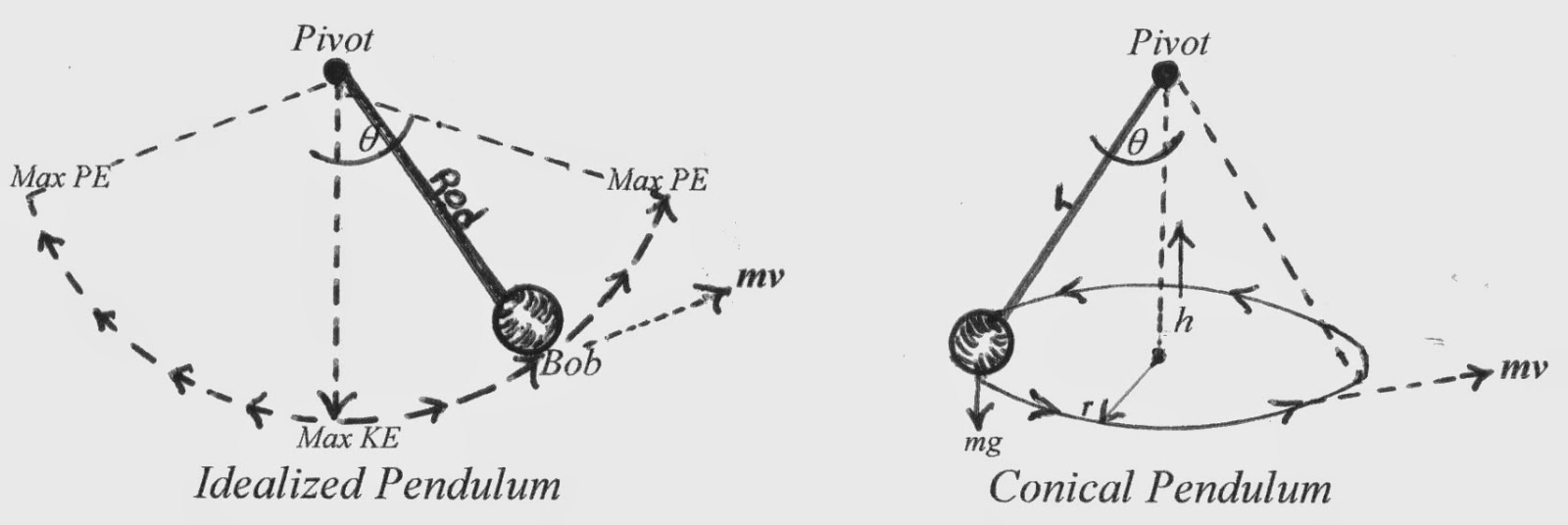FIGURE 2 - Pendulum Motion and Momentum Conversion

The Gyroscope

Another common device which appears to violate the laws of motion is the gyroscope. If a force vector is applied to the axis of rotation of a gyroscope operating at speed, the action becomes translated not to an opposite and equal reaction, but rather to a reaction that manifests itself at right angles (90°) to the direction of applied force; i.e., gyroscopic precessional force. The 90° deflection of the force (q ) appears to be an asymptotic limit for the gyroscope (and one that is apparent in other devices), and higher rotation speeds do not deflect the applied force beyond that value. This is a phenomena that is also seen in flywheels at high rotation rates.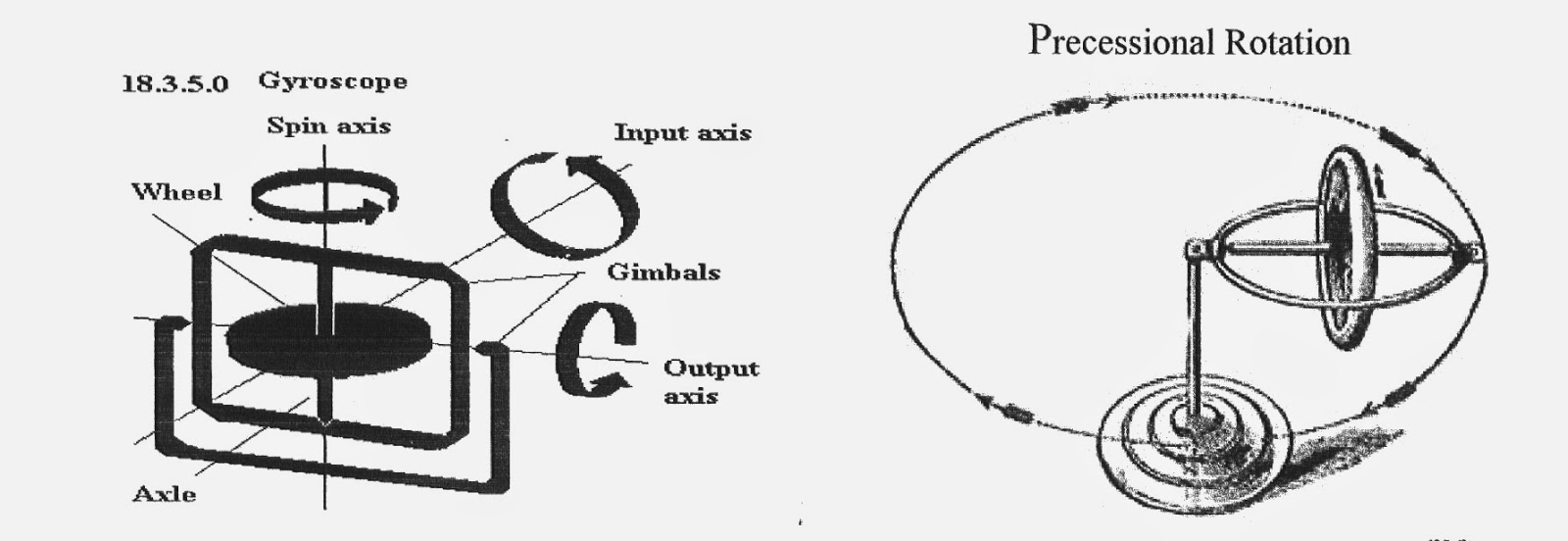FIGURE 3 - Translation of Force vector in a Flywheel/Gyroscope - IAW the right hand rule of gyroscopic precession, applied force (Input / mg) is translated into momentum at right angles to the plane of rotation and force (Output / precession).

Force Precessed Gyroscopic Devices

If two counter-rotating gyroscopes are linked together so that they form a single system, with the gyroscopes separate but free to either move apart, towards each other, or pivot at angles on their axis’s, and then two opposing forces are applied to move the gyroscopes, i.e., springs, magnets, solenoids, cams…, the dual gyroscope system translates the opposing forces into a unidirectional force at right angles to force application IAW the right-hand rule of gyroscopic precession (as in Kidd‘s Force Precessed Gyroscopes- see Fig. 4 & 5). This phenomena is different than the effect of shifting the cm in a closed system because the cg of this system remains constant (between the gyroscopes) and it is the two opposing forces that are translated into a combined precessional /
directional motion/force.FIGURE 4 - Dual Counter-Rotating Gyroscopes (Phenomena testing) - Springs drawing gyroscopes together/tilting produce unidirectional precessional force; magnets, solenoids, or cams may be used for the same purpose.

If two 180° opposed counter-rotating steel flywheels are spun on a secondary axis and an electro-magnet is positioned above and between the gyroscopes, opposing magnetic impulses can be delivered to the axis’ of rotation through the masses. This should have the effect of producing linear precessional impulses and a robbing some of the angular momentum for conversion into linear momentum via the magnetic impulses. (see A. Kidd, US Patent 5024112A, 6/18/1991 and Fig. 5.).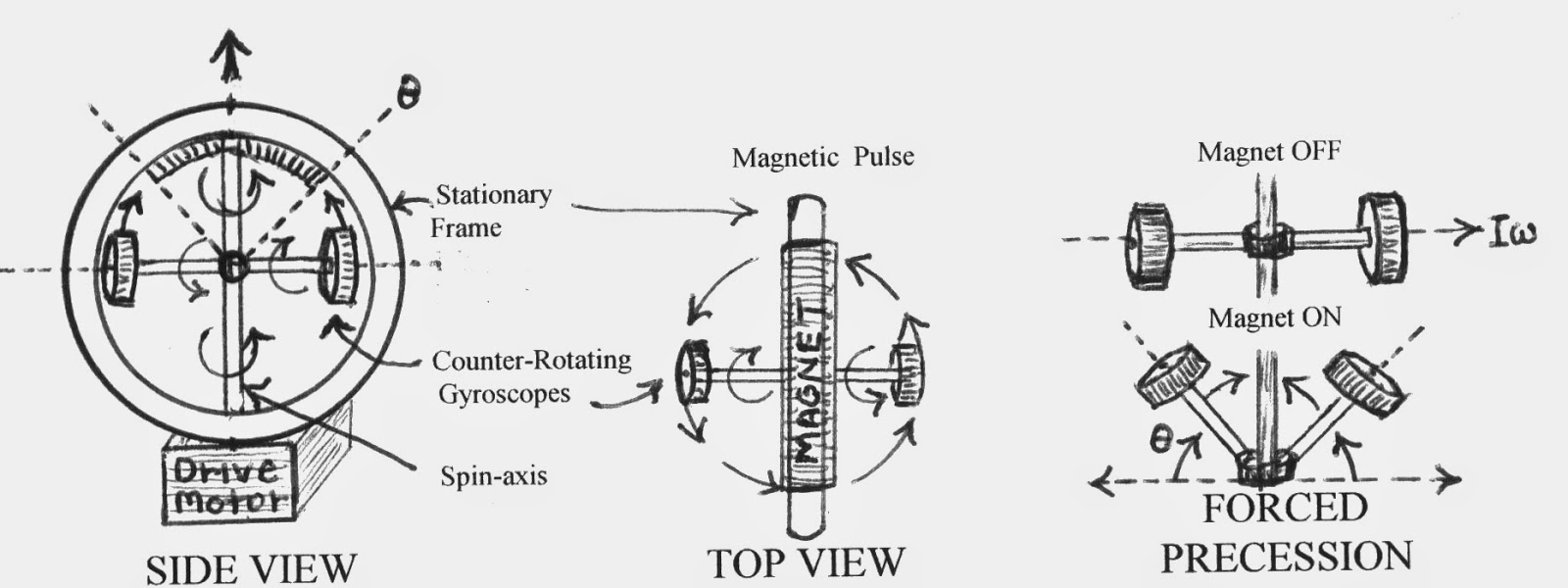FIGURE 5 - Force Precessed Gyroscopes - Simplified schematic of Kidd device but using magnetic Force instead of mechanical). Centrifugal action on the gyroscopes acts as a restoring force countering the magnetic impulses.

The dynamics of even a single gyroscope are quite complex and those of gyroscopic systems, such as those above, can be so complex and potentially confusing that building them may be easier than describing them mathematically. This is where trial-and-error experimentation comes in, and it may be more advisable to explain experimental results after-the-fact and with real data than trying to predict results mathematically; which may appear to violate fundamental concepts of motion and momentum conservation and for which Newtonian mathematical theory may only lead to the null hypothesis.

Rotational to Oscillating Circular and Bidirectional Motion
If a rotating flywheel is placed upon a frictionless table and has its center-of-mass (cm) displaced from the axis of rotation, the flywheel is unbalanced and at low speed will oscillate in a circular fashion about the center of gravity (cg); with the rate and magnitude of oscillation corresponding to the rate of flywheel rotation and degree of cm displacement. Therefore, the greater the displacement of the cm, the greater the out-of-balance condition and oscillation. However, in this form of forced harmonic motion, the cg of the system can be seen to lag behind the axial driving force by a phase angle φ. Even at low rotation rates, such an oscillating system can be made to achieve apparent unidirectional motion if the driving force of the system is varied in synch with the fundamental frequency of the system and slip-stick friction factors are considered [as anyone can testify who has slid themselves across the floor on a chair by suddenly shifting their weight]. However, when the rate of rotation of the unbalanced flywheel is increased, the actual oscillation/displacement of the whole system starts to dampen and can virtually be extinguished at very high rotational rates due to the inertia of the system and gyroscopic stabilization; i.e., the system is unable to translate the inherent inertial forces into actual displacement due to the rapidity/rate of change in direction of the forces, thus φ can be seen to grow to a maximum value of 90°. The result of such a dampening effect is that the actual center of gravity of the system appears to decouple from the center-of-mass, and the rotating flywheel tends to center itself around the torque force, while the virtual center-of-gravity (of the system) continues to oscillate.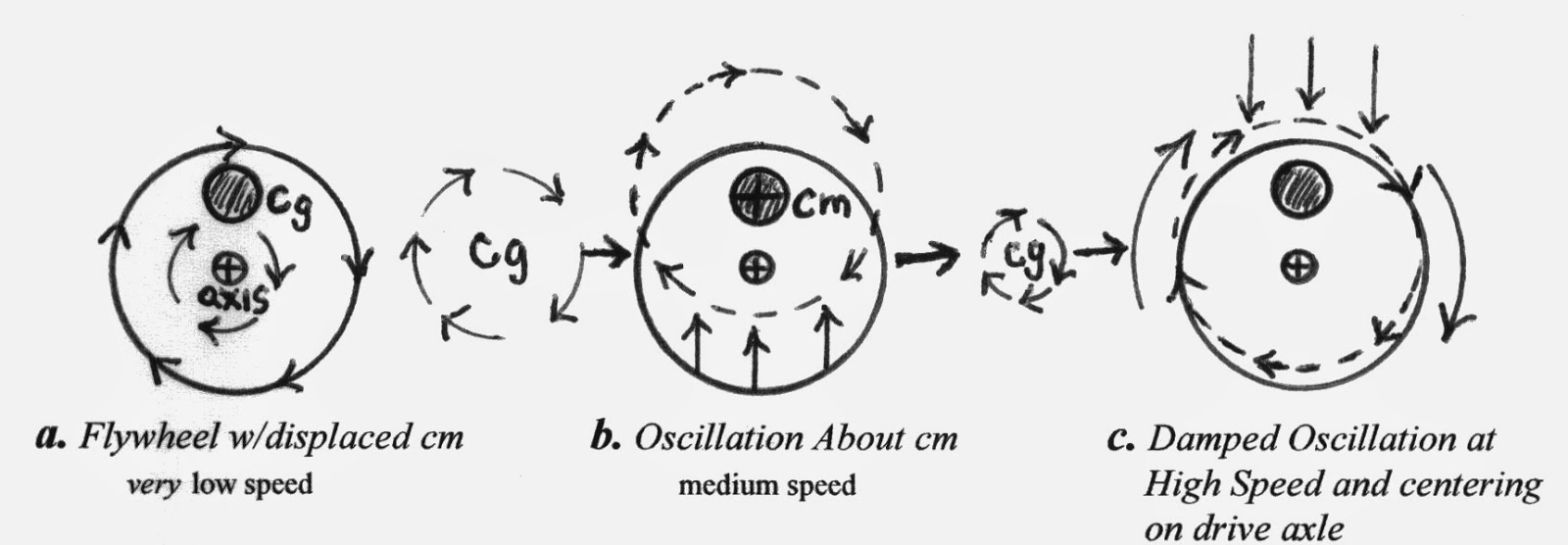FIGURE 6 - Oscillation in an Eccentric Flywheel -  Note c., angular momentum about the axis has a tendency to center the mass about the rotational axis. φ Iw> mv

Further, if two counter-rotating eccentric flywheels are linked as a single system where the actual center-of-gravity of the system lies between the cg’s of the two flywheels, the oscillation of the system is transformed into a bidirectional motion that manifests itself tangentially to the masses and through the system cg travel line (Fig. 7). This bidirectional change of cg (forced harmonic motion) resembles the accelerated motion of a loaded oscillating spring (accelerating and decelerating bidirectional motion) and can be described by the inclusion of a spring constant (k) in the motion equations (see Discussion). Further, like the dampened oscillation of the single eccentric flywheel at high rotational rates, the actual (bidirectional moving) cg of the dual-system is also decoupled from the cm and dampened at higher counter-rotational rates, while the virtual cg continues to oscillate bi-
directionally.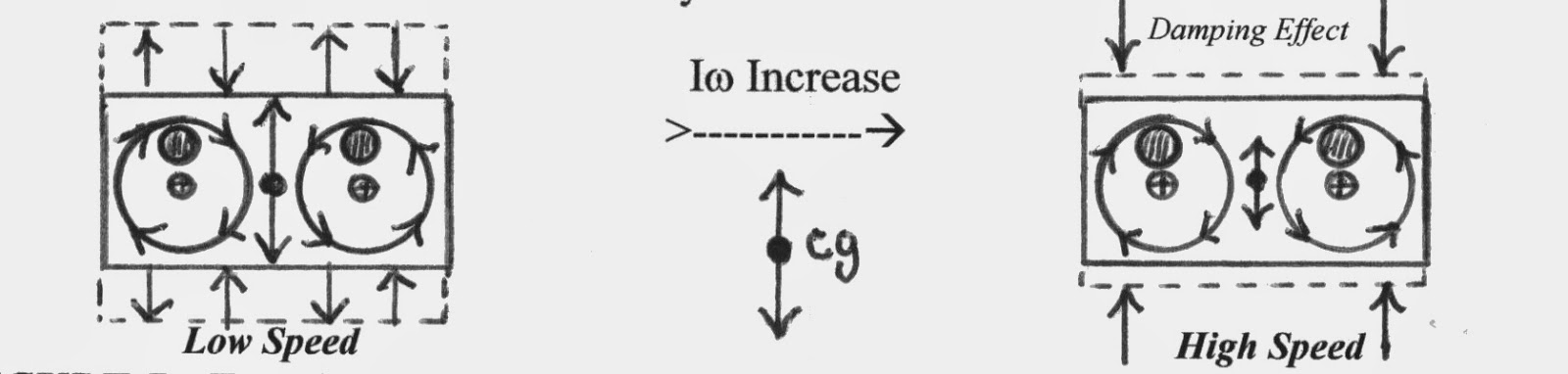FIGURE 7 - Two Counter-Rotating Eccentric Flywheels - Rotary oscillation is cancelled by counter rotation of masses, and bidirectional oscillation is dampened at high speeds and manifest as stress force at the axles. Counter rotating flywheels may also be stacked one on top of the other.

Counter-rotating eccentric mass devices, such as the above, usually have very limited application (they tend to shake themselves apart or produce unacceptable vibrations); but when used as mechanical oscillators (called Buehler Drives), the bidirectional impulses they produce can be harnessed to set up truly massive resonant/ harmonic oscillations, and have been used to simulate earthquakes when attached to large structures (if the rotational or oscillation velocity of the device is matched to the fundamental harmonic frequency of the structure).

Also inherent in this dual counter-rotating mass system is a phenomena that depends upon the direction of the converging counter-rotation of the system and is manifest as a small net transient impulse (surge / precession?) delivered along the line of travel of the cg and in the direction of convergence rotation; and this impulse may be amplified by modifications to the system that enhance the effect.

Rotational to Unidirectional Motion
Although the effect of forces acting on a balanced rotating flywheel/gyroscope are hard to characterize mathematically, it can be simplified by viewing angular momentum instantaneously and as an infinite series of opposite but equal inertial vectors that are manifest tangentially to the direction of rotation of the spinning flywheel/gyroscope. However, if we consider the unbalanced flywheel with a decoupled virtual and actual cg, and then induce cyclic perturbations in the cg, we find that the stage is set for loopholes in the 3rd law of motion because of the inability of any system to completely absorb or respond to rapid inertial changes. Thus many rotating systems can be made to manifest net unidirectional force/motion in apparent violation of the Third Law of Motion; these apparent violations are, however, just transformations of one form of momentum into another (angular to linear) and re-vectoring/deflections of the reaction to applied forces (as in gyroscopic precession).

Dean Drives - Mechanical Phasing [FHM]
One way to achieve the above effect is to mechanically link and unlink an eccentric counter-rotating mass-system to a secondary but encompassing subsystem during the cm convergence portion of the rotation cycle, and in such a way that both systems become one during the linked portion of the cycle and separate systems during the unlinked portion. This type of system is rather finicky and operates on purely phased mechanical inertial forces, but has been demonstrated by many patented working models such as the Dean Drive and Hampton’s Inertial Engine (ref. Dean, 1959; Stine, 1969; Harrington, 2011) Upon examination, I believe some of the more obvious net effects that have been demonstrated in these devices may be manifestations of slip-stick friction, although some (the quoted models) seem to truly demonstrate inertial drive effects.

Magnetic Coupling in Buehler-Type Drives [FHM]
Rotational angular momentum can also be transformed into unidirectional linear momentum by utilizing a dual counter-rotating eccentric mass system that is magnetically coupled and decoupled to the to the non-rotating part of the system during a portion of the flywheel's rotation cycle; so that a net impulse is created in the whole system and in one direction - much like Dean-type devices. At high speeds the opposite reaction impulse can be split and manifest as force vectors at a phase angle (j ) to the net linear impulse, thus producing a net opposite reaction. Optimum coupling/ decoupling to achieve a maximum net impulse can be obtained by experimentation, but probably lies somewhere around 1/2π Rad of the rotation cycle during cg convergence and at the bidirectional cg centerlines. By using non-magnetic plates (e.g., aluminum) with iron plugs or permanent magnets at their periphery to cause the out of balance / eccentric condition, and by minimizing the loss of angular velocity/momentum during the cycle, the magnetic translation effects can be further isolated, amplified, and directed. Such a device can be configured as stacked counter-rotating plates with the same offset cg’s, and can also be setup as a permanent magnet motor using electro-magnets to both rotate and cause variable momenta during the rotation cycle via an adjustable commutator. The weights in the platters can be exchanged for magnets (permanent or electro-) to further enhance the angular momentum and effects.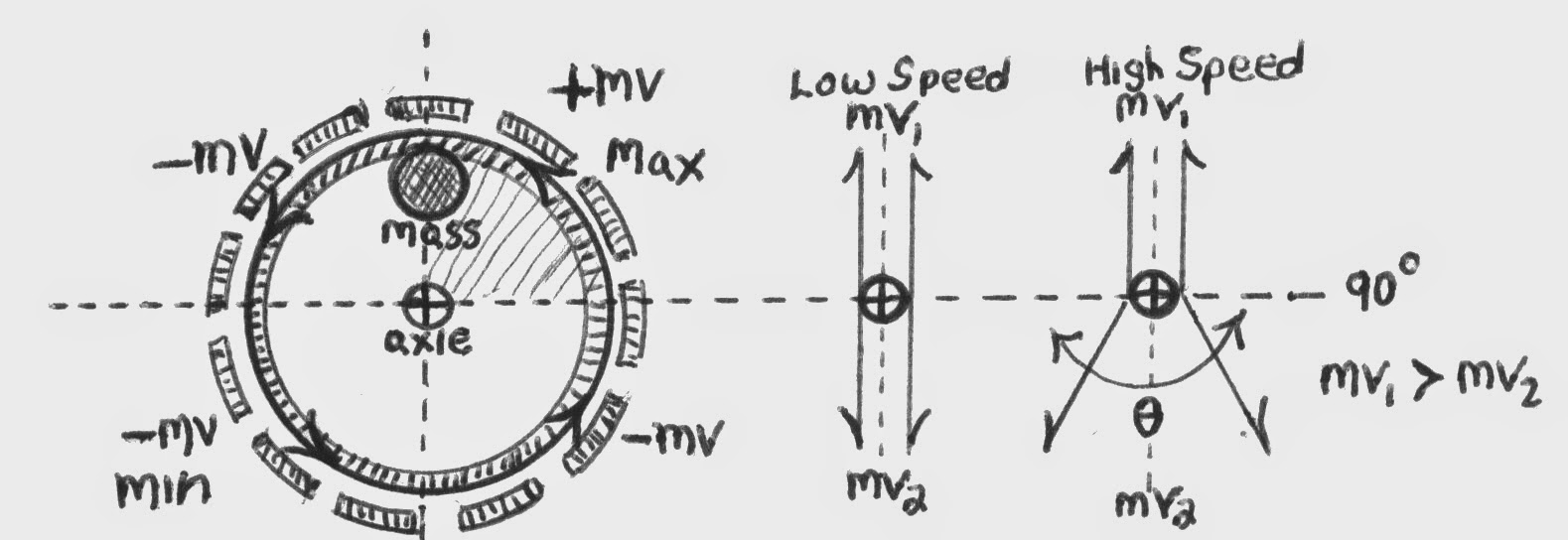FIGURE 8 - Stacked Eccentric Counter-Rotating Plates - Permanent magnet motor type system. Magnets can be used to both accelerate and decelerate mv at opposite poles to create off-set Iw /mv. Permanent magnet motor can control rotational cycle and perturbations via an adjustable commutator.

Centrifugal Devices

Another means of translating rotational to unidirectional momentum has been demonstrated by utilizing horizontally mounted counter-rotating platters with free-swing weights attached off-center by eccentric pivot points. If the platters are rotated at a proper speed for the system, the weights are flung outward and then continue swinging and are drawn inwards by the angular momentum of the platters (working in conjunction with the eccentric pivots) towards the platter’s centers. These free-swinging weights are made to undergo one orbital revolution per rotation of the platters (see Figs.9a & 9b). According to the inventor, the counter-rotation of the platters negates all forces except a unidirectional one (Gilbert, 1985). The unidirectional force produced by this device depends upon an offset and increase in momentum during the outward swing; i.e., variable momentum (Iw) over the course of the orbit. Such a system may not work effectively (or at all) at high rotation rates, as the weights would be unable to respond to the rapid inertial changes in their eccentric orbits, thereby limiting high performance applications. However, this device does demonstrate in a simple and elegant manner the principle behind other more complex inertial drives.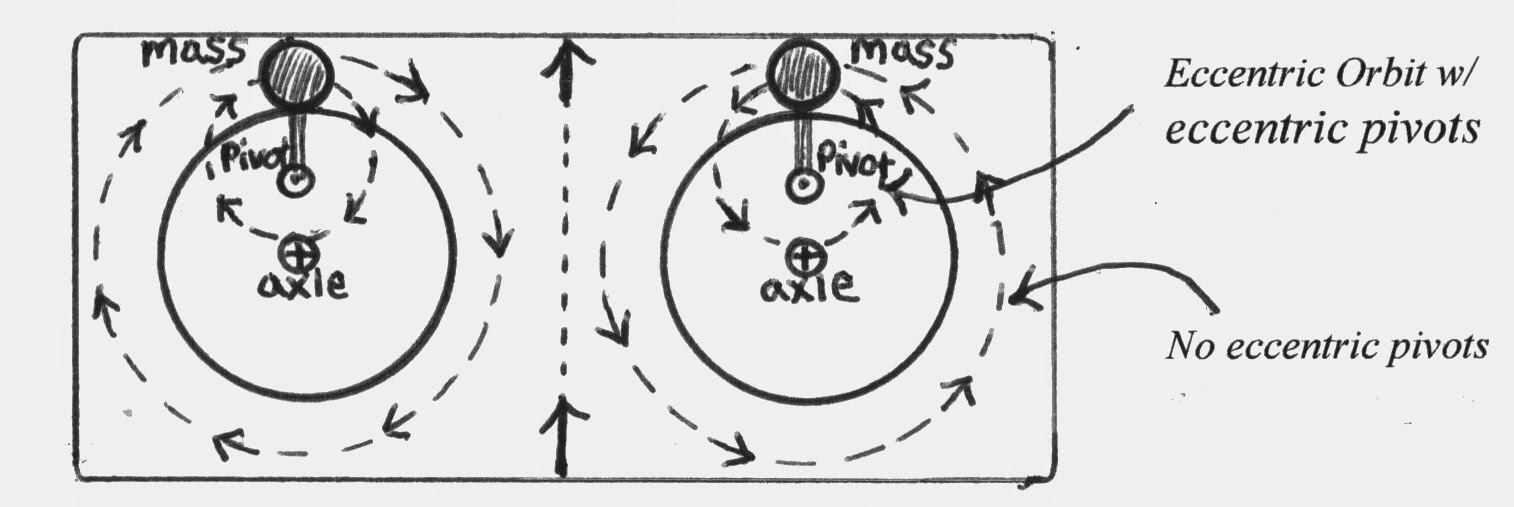FIGURE 9a- Counter-Rotating Platters With Free-Swinging Weights & Eccentric Pivot Points to Control Weight Orbit.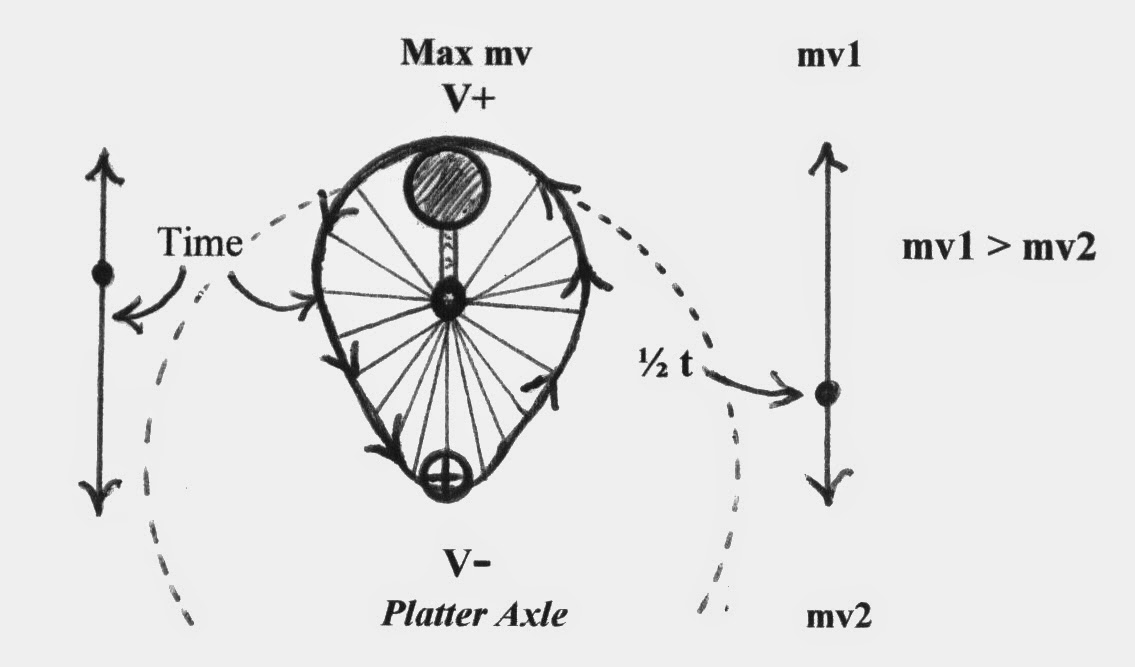FIGURE 9b - Centrifugal Time & Momentum Rotational Diagram - This describes the travel path of the rotating mass (displaced cm) over time and in relation to the main drive axle in the centrifual system.

Fig. 10a is a redesign (Renner) of the above device (and its working principle) to eliminate the need for pure centrifugal force in swinging the weights outward; instead, using forced harmonic motion (FHM). In this device, gearing is used to rotate added eccentric weights/discs in synch with the main platters. Fig. 10b is a time vs. offset mass positional diagram, showing the changes in momentum in one rotation.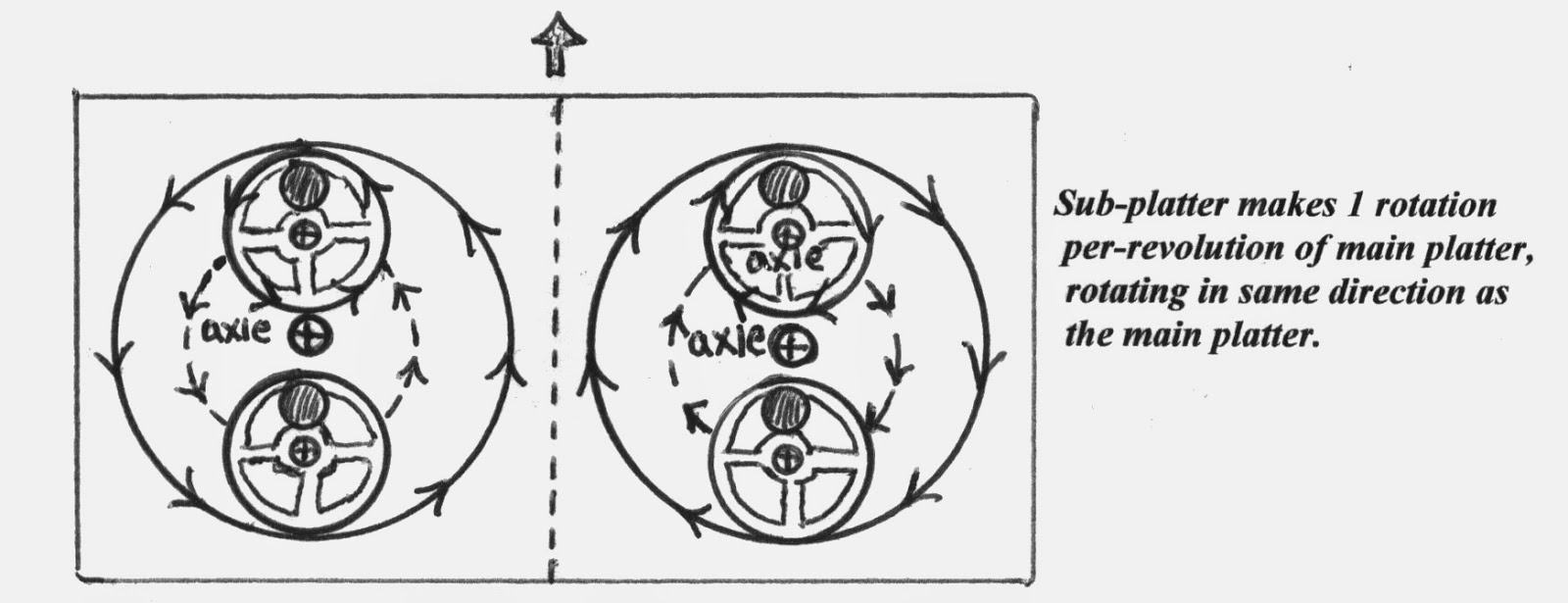FIGURE 10a- Counter-rotating Platters w/ Off-set, Rotating Eccentric Sub-platters Used to Take the Place of the Free-swing Weights. Multiple geared sub-platters are used to mimic the centrifugal action of Gilbert device. Although outward throw momentum is reduced, this is Forced Harmonic Motion and not limited to low or specific speeds.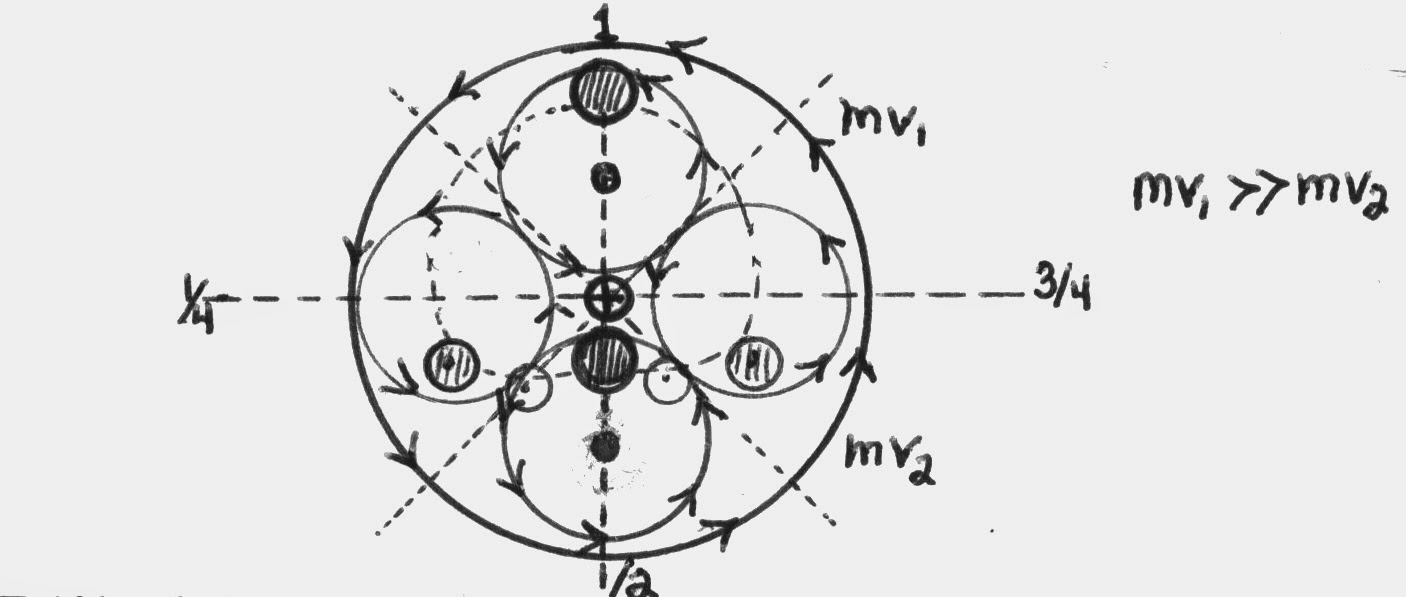FIGURE 10b - Orbital Positions of Sub-Platter Masses - Note significant offset of net momentum/mass. One to four+ sub platters can be used with very little re-engineering.

Because of the large amount of energy stored in high speed flywheels, this type of system may represent the best interim device for demonstrating and creating a functional inertial drive by mechanically low-tech means; that is, until the phenomena can be further amplified by more high-tech means; i.e., plasmas and phased electro-magnetic induction.

Magnetohydrodynamically Driven Liquid and Plasma Inertial Drives
Directional inertial drives can also be created by forcing conductive liquids (i.e., mercury, superfluid ferrofluids) or plasmas to counter-rotate in tube raceways that are geometrically shaped to create differential momentum via the Venturi Principle. Propelling the liquid or plasma in such a device can be done by magnetohydrodynamics, so that the only moving parts are the conductive liquid or plasma. Total momentum is conserved within such a system, but directionality is not; i.e., action momentum is more-or-less linear and central while reaction momentum is distributed as a series of radial vectors in multiple directions along the larger curve. Multiple tube arms may be used in such a device and other mag-drive coils can be used to steer/alter the action directionality of the device. The following schematic is of a two-arm device:FIGURE 11: Liquid Metal or Plasma Inertial Drive -  Note that the geometry of this device resembles an ovoid magnetic field. Ultra high-velocity metal plasmas interacting with electro-magnetic propulsion (Lorentz Force) systems may be the first step towards more advanced inertial or warp drives. Or, perhaps high gauss electro-magnetic fields can be manipulated thru varying strength, directed flux, and pinching to have a similar effect.

DISCUSSION

Prior to this point I have neglected to include the mathematical principles of the devices I have discussed. This is mainly because most accepted mathematical descriptions are essentially tautological, conforming to accepted laws and principles of motion and momentum; thus useless in describing any new concepts that would violate those principles; i.e., “mathematics will lead you only to the logical conclusions of your basic assumptions” (Stine, 1969). However, there are mathematical transformations of accepted laws (hard won from engineering experience) that can explain the discussed phenomena, and in this section I will discuss these proposed motion law transformations as well as discuss some of the implications of inertial drives when used as impulse engines or possible warp drives.

The basis of unidirectional impulse from angular rotation (discussed in the previous sections) has best been described by theories invoking the concept of the Critical Action Time; a concept conceived of (directly or indirectly) by many physicists of excellent reputation and credentials; i.e., William O. Davis, Hermann Von Schelling, Henri Coanda, Serge Korff, Gilbert Plass, and several others (including Werner Heisenberg). The development of the theory, however, can be credited directly to W.O. Davis (1962a,b, 1967), who worked out the mathematics of the phenomena based upon real-life engineering experience and modification of the Newtonian equations/laws. The general principle of the critical action time (CAT) is “that the energy of a system cannot be changed in zero time”, or, “that there is a time in which a system as a whole cannot accept energy (input)”. This concept essentially says that whenever the rate of energy input into a system is too great for the system to absorb, the excess energy must either be excluded or leave the system; either by translating the energy to another system and/or changing the form of the input energy so that it may be radiated away (Davis, 1961, 1962,1967). Davis’s revision of the basic Newtonian equation for applied force in one direction opened the door for a theoretical inertial drive effect by: 1) adding a third derivative containing the critical action time (D), 2) including Hook’s spring constant (k) in the starting condition, and 3) including a viscous damping coefficient (V) in the first derivative; the revised force equation and n=3 summation is:

F = kx + V dx/dt + m d2 x/dt2 + Dm d3 x/dt3

The implications of this Newtonian revision (Davis Mechanics) is that “There is a force proportional to the rate of change of acceleration as well as Newton‘s force proportional to acceleration itself”, (called surge: Davis, 1962); thus in systems with cyclically unbalanced forces and subject to harmonic driving forces, a unidirectional force can manifest itself through induced motion of the axle(s) - ergo, an inertial drive.

Space Drives & Space Warps
What can we expect if an advanced inertial space-drive system was created and what actual effects would we experience if theoretical velocities of near-light-speed (or beyond) are made possible? To create a true interstellar drive, space itself would have to be warped/foreshortened in the direction of travel without involving the space vehicle or its’ passengers in the consequences of relativity (internal momentum). This may be possible by experimenting with the various manifestations of momentum and the actual effect they have on the fabric of space-time. Cutting to the chase, it may be possible to create a asymmetrical “warp” in the fabric thru forced harmonic motion and perturbations of momentum in high speed angular acceleration.
In Einstein’s Equivalence Principle, the (apparent) force of gravity (mg) is the same as the force produced by linear acceleration/deceleration (mD v) and angular acceleration (ID w ). But he also believed that gravity was a phenomenon created in the fabric of Space-Time, where mass warped or proportionally distorted this fabric rather than being an actual attractive force mediated by gravitons. Therefore, if the force of gravity creates a 4d warp in space-time, this means that the forces of linear acceleration, deceleration (ma /-ma), and angular acceleration (ID w ) also produce warps in space-time if they are in fact equivalent to g. In the following diagrams, deceleration is viewed as two possibilities; one as a positive warp ahead of the decelerating object, the other as a negative warp behind it (negative acceleration). The conceptual difference between these two possibilities may be quite significant in being able to form a propulsion warp effect.FIGURE 12 - Space Warp Gradients Produced by Mass, Linear Acceleration, and Deceleration/ Negative Acceleration.

Angular acceleration can be viewed as a unique g warp case, but its attractive Fg effects can be tested for by duplicating Cavendish’s experiment for deriving the Gravitational Constant (G), but using a high speed flywheel rather than a second large mass. With this modified experiment, any significant virtual mass increases could be determined by measuring potential g gradients at different positions around the flywheel.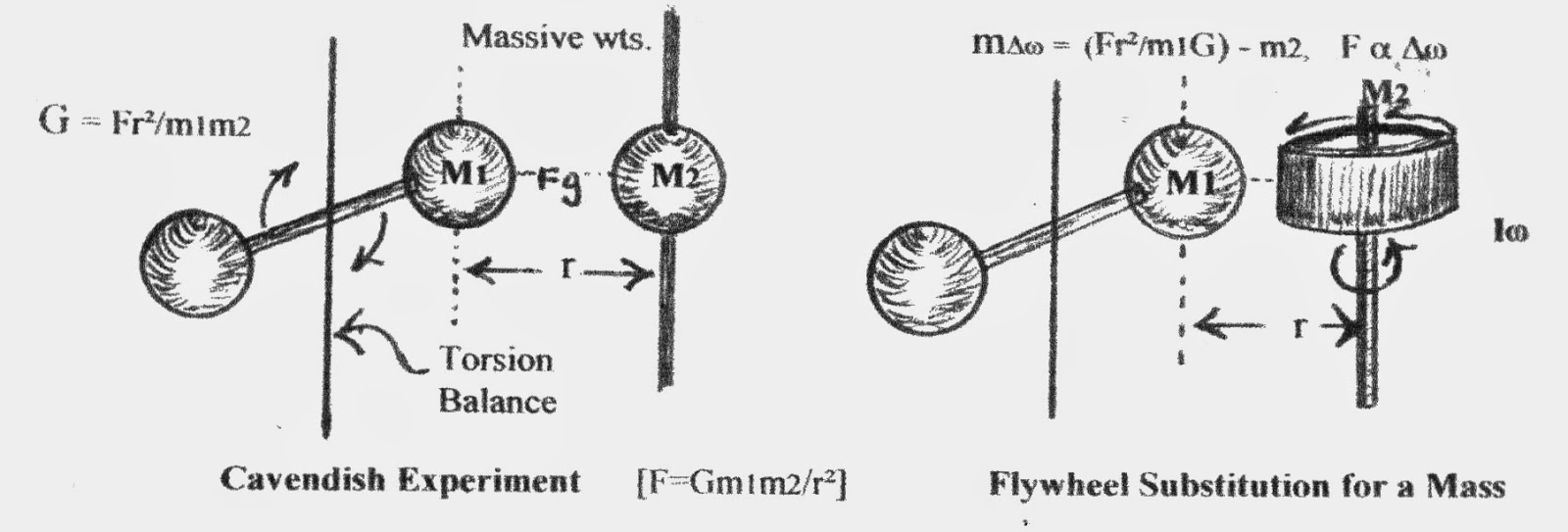FIGURE 13a - Substitution of a Flywheel for Mass in The Cavendish Experiment

If there is increased attraction between the flywheel and the torsion mass as the speed of the flywheel is increased, then it may be possible to harness this phenomena (via displaced virtual cgs and offset momentum) to create a warp ahead of a space craft for it to “fall“ into; i.e., directional movement without actual action/reaction propulsion.

Figure 13b is a diagram of angular momentum/ outward g force as it increases from the axle to the rim of a gyroscope/flywheel at constant angular velocity [F(Iw ) = r /r² ]. In it you see 0 g force at the axle and then an exponential increase in g force to a maximum at the rim. Beyond the rim, g force again drops to zero, but does all the “attractive” g force also drop to zero?; i.e., if g forces create a dimple in the space-time fabric, is there any dimple gradient beyond the rim edge? The above modified Cavendish experiment should be able to test this theory. Perhaps by creating displace cg’s (virtual cg’s) thru variable momentum we can create such a dimple gradient and make a useable warp in space-time.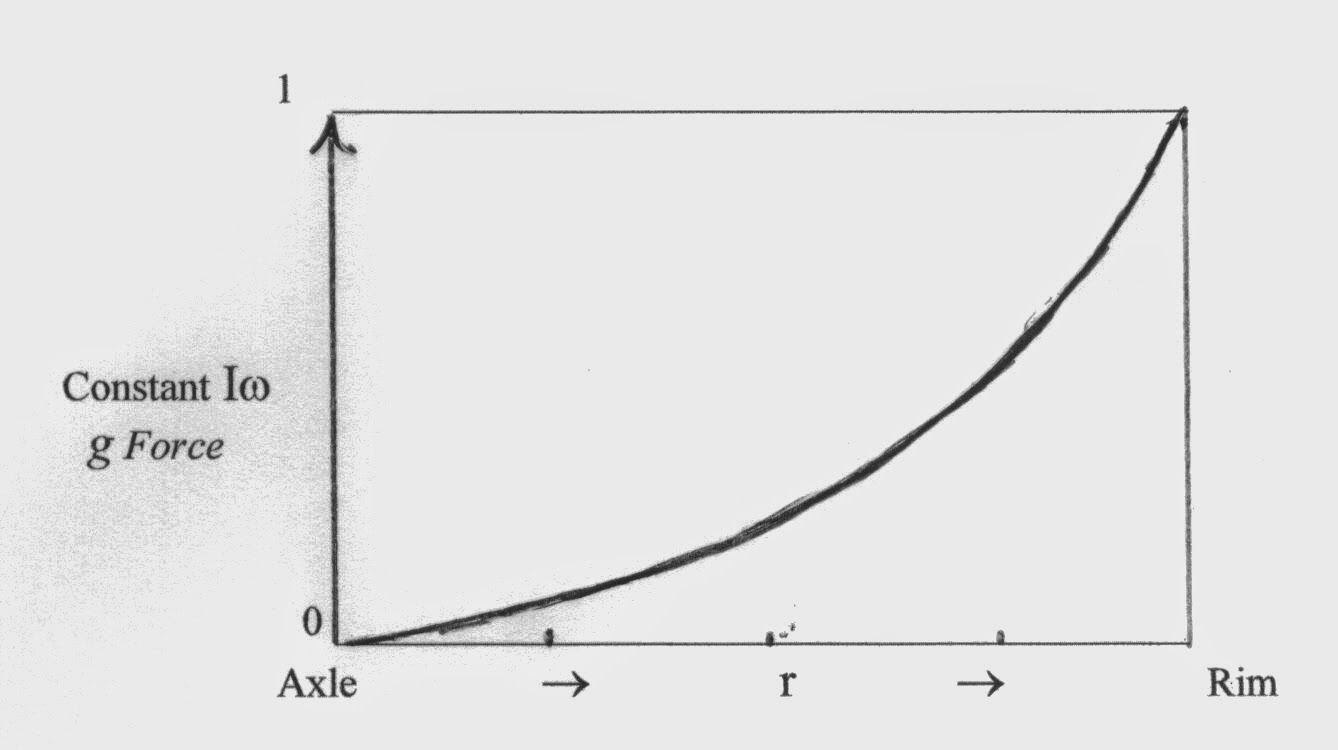FIGURE 13b - Increase in g from Axle to Rim in a Flywheel at Constant Velocity - r = distance from axle; Iw = angular momentum r(g force).

The second figure (14b.) in the following diagram is a space-time warp proposed by the Mach Principle / Woodward Effect and Alcubierre’s theoretical warp engine. However, angular acceleration devices like that proposed in Figs. 10 & 11 could also create this kind of space-time warp.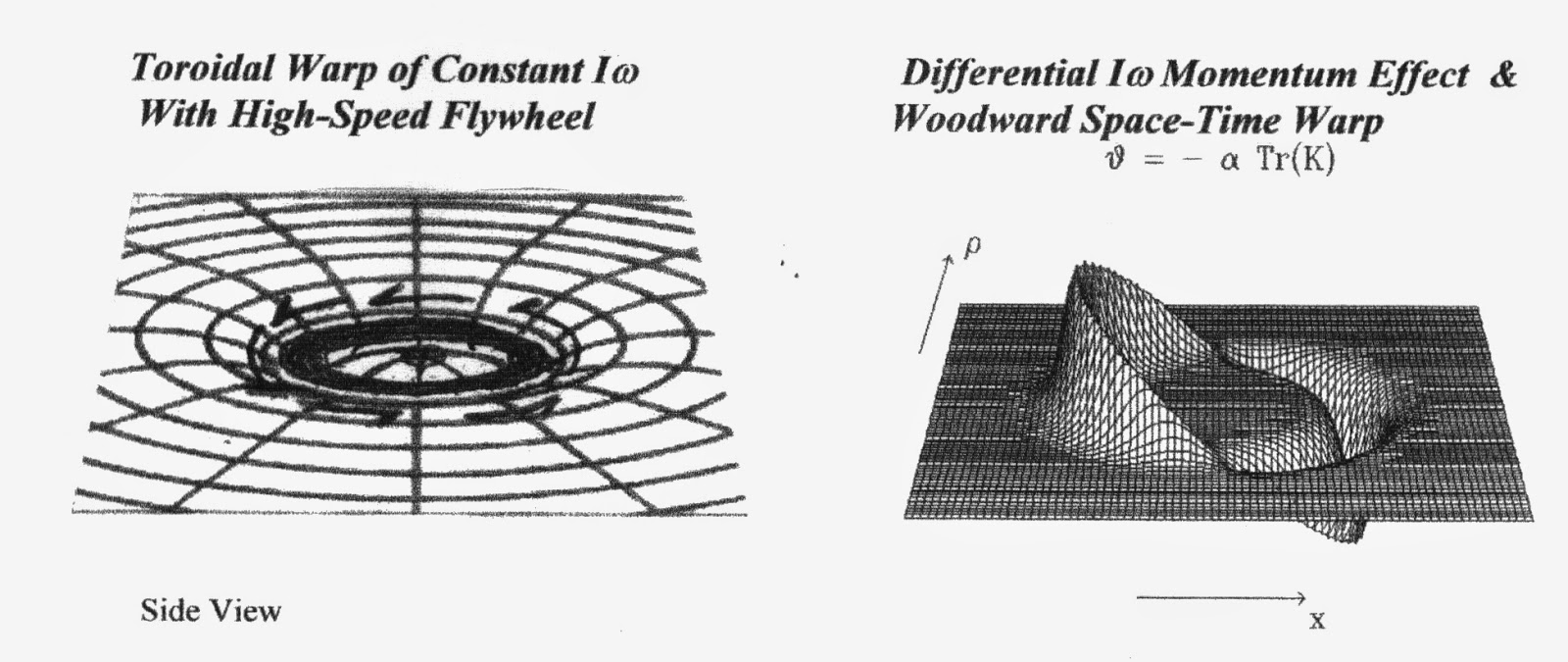a.                                              b.
Figure 14a&b - Angular Momentum & Proposed Space-Time Warp Effect of Off-Set Momentum Device (see Fig. 10) Note that the g effects drop off as the center of rotation is approached in both effects. Also note that the Woodward Effect warp favors a negative acceleration interpretation of deceleration momentum.

In Figures 12 & 14, the effects of mass / acceleration on the fabric of space-time (Higgs Field?) act as if space-time were a non-trivial medium that was creating g drag on an accelerating mass and producing an effect like the wake or bow wave of a boat; or, as if the inertial mass was being attracted towards some dimension beneath a space-time interface / the Higgs Field. The effects of deceleration (negative acceleration) on this fabric seem to be the most telling and may demonstrate an actual negative g force / space-time warp effect.

Electromagnetic, Magnetic, and Gravitational Attraction
If gravity and acceleration warp the fabric of space-time, what about electro- magnetism and magnetism? We know electric charges add to the mass of a particle, and that magnetism as a force behaves similarly to gravitational force and can be alternately described by substituting the strength of two magnets for the masses in Newton’s gravitational equation. However, unlike gravitational force, magnetism and electro-magnetism have a repulsive nature depending on magnetic polarity. So, is it feasible that magnetism and electromagnetism also create a warp in space-time /the Higgs Field via electro-magnetic induction forces (i.e., Lorentz Force)? And, under specific conditions, might gravitational force also have a repulsive nature, perhaps as a property of dark matter or negative acceleration?

Fg = G m1· m2 / r² ~ F(-a) = G m(-a)· / r² ~ ± F = A ± mmf · ± mmf / r² [mf = N or S]

Faster-Than-Light vs. Wormholes
Recent experiments have supposedly “accelerated” photons to velocities measured at over 300 times c, demonstrating that the speed of light might not be an upper limit to velocity. However, as photons are supposed to be mass-less, but have momentum, perhaps these photons may have simply had their relativistic “mass” increased by forcing them to exceed their natural top velocity, causing them to worm-hole/foreshorten the distance traveled so they only appear to exceed light speed. Or, perhaps the speed of light is not the ultimate speed limit in the universe, but only the velocity limit of the normal space-time universe. There have been proposed theoretical quantum “particles” that travel beyond the speed of light, i.e., tachyons or neutrinos with tachyon nature; which would have space-like rather than time-like four-momentum. In the tachyon dimension, deceleration theoretically produces acceleration of the particle, almost as if that dimension was a mirror image of our own 4-dimensions. Nonetheless, these photon experiments could be the possible proof of a worm-hole effect if their results are reinterpreted using Davis Mechanics and General relativity, and by seeing if the photons actually did have an increase in relativistic “mass“. Thus, if the speed of light is an absolute constant and unable to be exceeded, then the foreshortening / compression of space-time via a wormhole would be the most logical explanation for apparent faster-than-light travel; (perhaps photons are just wavicles in/of the Higgs Field?).

According to the Theory of Relativity, when a non-zero mass particle (or a spaceship) approaches the speed of light (c) it experiences an exponential slowdown of time and a contraction along its’ direction of travel. As the speed of light is closely approached, internal clock time in a spaceship would slow to zero and the direction of travel distance would also appear to shrink towards zero; plus it would require an infinite amount of energy to actually achieve c because of the exponential kinetic mass increase. Be that as it may, at c, an observer (in the ship) would not experience any passage of time, and distance in the direction of travel would appear to be zero; so they would essentially travel to the end of space-time at the instant c was reached. Such an object would also experience an exponential increase in momentum energy towards the infinite (that is, according to Lorentz transformations in Relativity theory). This is where I believe the Theory of Relativity may break down, and may not actually represent reality at c or at hypothetically greater (superluminal) velocities. In theory, the warp created in space-time when trying to reach c might actually create a bubble outside of normal space-time, and not be subject to the limitations imposed by relativity theory; i.e., time dilation, continuous and exponential relativistic “mass” increase, or internal g forces.

Relativity and The Mass-Velocity Barrier
According to conventional relativity theory, before any non-zero mass object could come close to achieving the speed of light and infinite kinetic mass, it would come up against the space-time curvature limit for massively dense objects; i.e., the creation of a black hole IAW General Relativity via Schwarzschild’s equation for the space-time curvature (Ω - see Fig.14 & 15):

rs = 2Gm/c2 à m = rs c2/2G.

Yet, since the objects’ mass/momentum increase is due to its’ velocity approaching c, it should be a vector quantity. Thus time-space curvature should also be a vector quantity in the direction of travel and create a worm-hole rather than a black hole. As the speed of light was approached, distance and time in the direction of travel would continue to contract. The closer to c the velocity was, the greater the relativistic momentum mass (r r) and contraction of space-time in the direction of travel, until it would approach infinite r , 0 d, and 0 t at c. Even still (acknowledging Relativity Theory), c would be any non-zero mass’s ultimate velocity limit, even though distance traveled per unit time would have the appearance of being faster than light; and this may be (again) an alternative explanation for the experiments where photons were (apparently) pushed to faster than light speed. So, does the Theory of Relativity breakdown at the speed of light and superluminal velocities, just as the laws of physics breakdown beyond the event horizon of a blackhole, or as Newtonian starting assumptions need to be amended at highr velocities even well before relativistic velocities are approached? The following diagram shows how velocity is limited by the speed of light while kinetic mass/energy continues to increase exponentially (with equivalent energy input); that is, until a space-time curvature limit is reached.Relativistic Mass/Momentum/Energy (exponential)

Figure 15 - Velocity and Relativistic Momentum Limits - Each graph point is a factor 10 increase in kinetic energy/momentum mass; which increases until the space-time curvature limit and production of a blackhole or worm-hole: PW = 2Gm/(v/c)²= Rc²/2G

Wormhole Effect
Relativity Theory predicts that momentum-mass would grow proportionally to the energy needed to propel the object to near light speed, i.e., m=e/(v/c)2. But what happens when the upper limit for mass/dimensional density is reached? Since the “mass” increase is due to linear acceleration/ relativistic momentum (a vector quantity), would we get a black hole or a worm hole? The following diagram is a modified Lorentz Transformation diagram reflecting the upper limits for mass density or relativistic momentum (ala Schwarzschild radius R):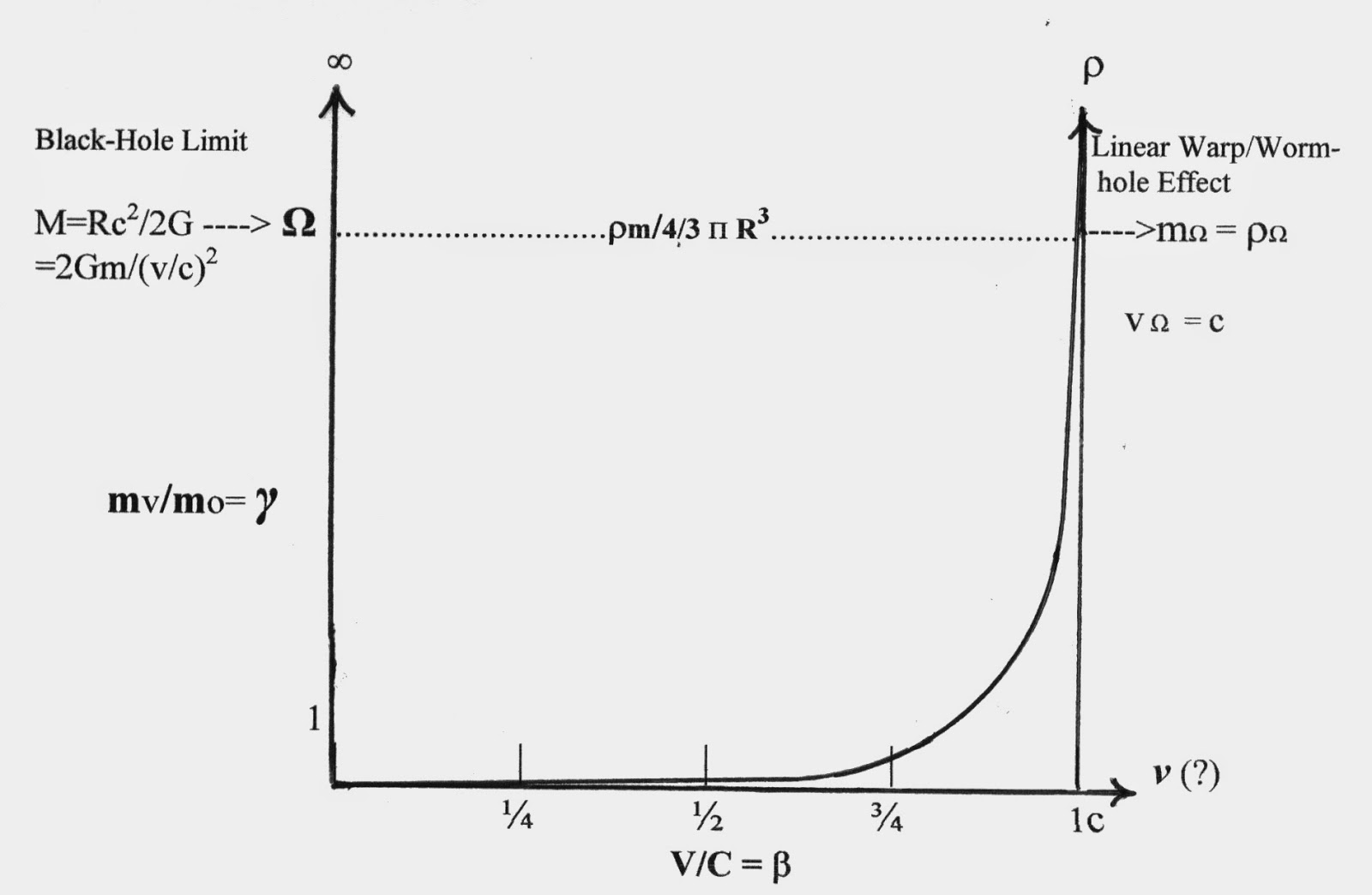FIGURE 16 - Modified Lorentz Diagram - Momentum/mass increases as a function of velocity (r) but encounters a limit (Ω) where a worm hole should be created. R= Schwarzschild radius, γ = Lorentz factor,b = v/c, mΩ = m/4/3 pR3= mass density limit, G=Gravitational constant, M/r= relativistic mass/momentum, m = moving mass, mo = rest mass, v = velocity, c = speed of light.

Lorentz Factor and Blackhole/Wormhole Effect (γ= 1/ Ö1- (v/c)2)
Relativistic “Mass” (momentum):
mr = m Ö1- (v/c)2 ----> r = mov/ Ö1- (v/c)2

Mass limit ® Black-hole or Worm-hole:
c = Ö2GM/R ---> vG = ÖGM/r --->vG = c ÖR/r

M = Rc2 /2G ---> mo = 2Gm/(v/c)2 ---> v = c Ö2Gr /mo
or: vΩ < c ~ Ö2G moΩ ---> moΩ = vΩ 2 / 2G » r W /4/3p R3

There also seems to be an interesting connection between mass and time within General Relativity and the Equivalence Principle. It would seem that they are inexorably linked as part of the four-dimensionality of space-time. Mass-less or virtually-mass-less particles that travel at (or close to) the speed of light appear to be timeless, that is, the moment of their creation is the same as their moment of extinction. From the perspective of a particle at c, there is no time nor distance/third dimension. Mass, on the other hand, almost always seems to have time and three dimensions associated with it (with the exception of a black-hole?). Thus time appears to be a basic property of mass within the normal space-time universe, describing its‘ interaction with a Higgs Field.

One problem I see in interpreting Relativity Theory is with the paradoxes that seem to occur at and beyond c. Paradoxes, however, can actually be seen as just the limitations or descriptive limits of the paradigms, not prohibited realities; as reality is what it is independent of a paradigm’s ability to describe or account for it. Therefore, when we encounter an apparent paradox, we probably need to re-examine and adjust the paradigm, not deny the reality. For instance, the Twins Paradox is not really a paradox if the twin who journeyed close to the speed of light did not return younger than before he left. Even transatlantic jet travelers and astronauts experience a slower passage of time compared to the earthbound and stationary. Relativity paradoxes are then just different comparative realities created in different inertial/mass reference systems.

Another problem is that of supposed causality violations and hypothetical backwards time travel. However, any particle, no matter how fast it travels, arrives at a distant location after it departed, not before. After all, photons don’t travel backwards in time (unless photon acceleration experiments can actually demonstrate this), and quantum teleportation is instantaneous and yet allowed without violating causality. Analogously, a supersonic jet or rifle bullet arrives ahead of it’s sonic boom or report sound, so who’s to say that a superluminal object can’t physically arrive ahead of its’ light and not violate causality, just appear to do so? After all, the light we see from distant stars and galaxies is what happened thousands, millions, or billions of years ago, not their present position / state of existence; so what we see is not what is, but rather what/when it was. Maybe there actually is some kind of universal reference field in the Universe (Higgs Field / Mach principle?), one created by the interaction vector sum of all mass/energy (M) in the universe acting on the mass/energy at a particular position in space-time (Ñ Fg = G Ñ ò mM/). This could be the equivalent of a space-time ether. Or, perhaps the field is a Higgs Field membrane interface with a dimension of dark matter and energy.

Conclusions
If we conceptually integrate Davis Mechanics and inertial drive experimental results with Einstein’s Equivalence Principle, the Mach Principle, the Higgs Field concept, and the Woodward /Alcubierre Effect /concept, we can see how virtual faster-than-light travel and warp drives may be possible. By viewing the space-time continuum as a 4 dimensional matrix that can be distorted/warped by mass, electro-magnetism, charge, and the creation of virtual mass (thru acceleration, ma & Iw), and allow for the shunting of space itself, we may find the really big loophole in Newtonian and Einstein’s physical laws that would allow for real-time interstellar travel. As a closing thought to ponder, what if we (hypothetically) consider the described performance and behavior of supposed alien UFO’s that have been seen for centuries… isn’t that exactly the kind of supposedly impossible flight characteristics we would expect from an inertial warp drive that doesn‘t rely on action-reaction propulsion?

The Time Quantum
The deterministic treatment of applied force and other revisions in the Laws of Motion (Davis Mechanics) allows one to calculate the smallest meaningful interval of time for any finite system (6.27x10- 24 s) a value also independently arrived at stochastically by Hermann Von Schelling (1963), from physical assumptions made by Gilbert Plass (1961), and from theoretical estimates by Werner Heisenberg (1956). This value is believed to be a universal constant that determines both the speed of light (c) and Planck’s Constant (h) - both of which can be derived from this time quanta or vice-versa using Davis Mechanics. By accepting the existence of a time quanta, we can now make sense of why certain constants are constant. For instance, the speed of light c (as a velocity) is a constant composed of distance per unit time (d/t), meaning that both of these factors or dimensions must vary in concert as a quintessential manifestation of the space-time continuum. A time quantum would mean that time (based on our concept of space-time) is not a continuous dimension, but rather one of fixed moments / instants separated by a mathematically infinite number of intervening instants or probability distributions. Is this perhaps (conceptually) how the multi-verse works? … Not as a continuum, but as 3 dimensional moments or instants (like frames in a motion picture), and where intervening non-moments are outside of our space-time frame of existence; yet the separated instants providing the retrospective illusion of a continuous 4th dimension.

The probability distributions between time quanta would likely resemble a sine-like wave, with 0 probability after one time quantum followed by a growing probability distribution until the next time quanta occurred, “fixing” that particular moment. This process would be driven by probability and fixed by entropy, meaning that backwards travel in the same time stream (arrow of time) would be virtually impossible because of the 0 probability time well after a time quanta instant.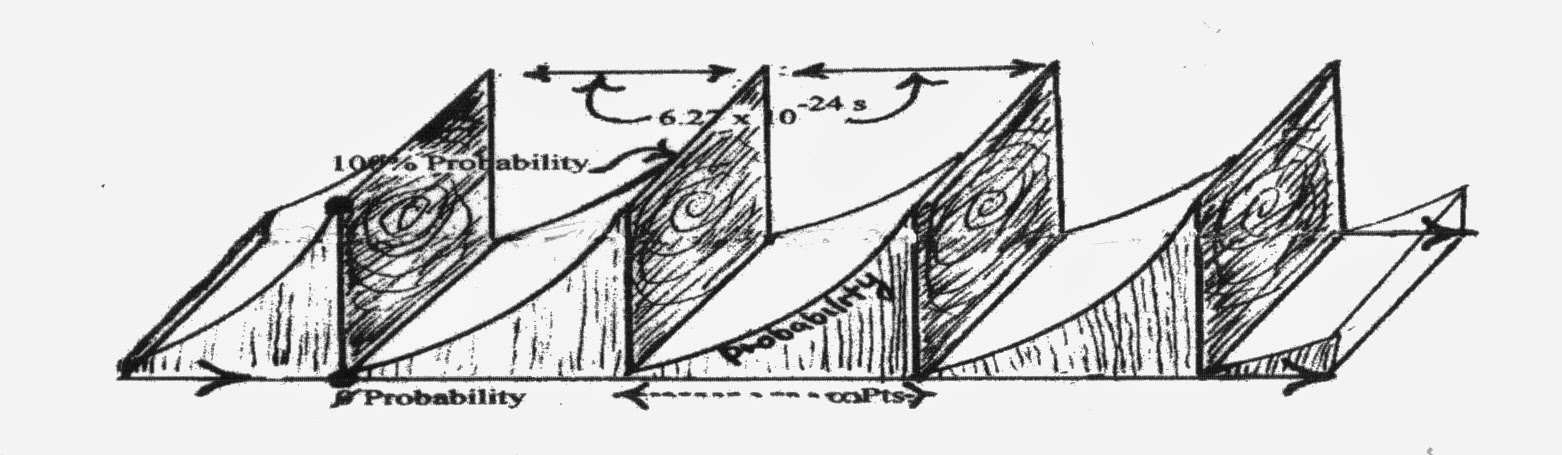FIGURE 17 - Space-Time via Time Quantum Perspective - 3D Time instants connected by sine-like probability distributions. Each instant is one retrospectively fixed, time-probability dimension of the multiverse. Between the instants would lie n-number of other probability instants defining other possible realities. Entropy provides directionality to the ‘arrow of time’ and backwards time travel attempts would be thwarted by the 0 probability time well.

However, if backwards time travel was possible, then any attempt to enter a time quantum instant that was already “written” (past) would have to create or graft on a new time quanta sequence/ time stream; thus creating an alternate and parallel universe in a Multiverse. This new universe would diverge the moment you entered it, and all changes you might make would have no effect on the sequential series of fixed events in the universe you left. That is, assuming that present and future events cannot effect or influence events of the past (as some Quantum Theorists have proposed). In this perspective, Hawking’s feather-of-time would resemble a series of connected tufts, with attempts at backwards time travel creating another fractal pattern in the tuft series (Multiverse). The following figure is an attempt to show this alternate view.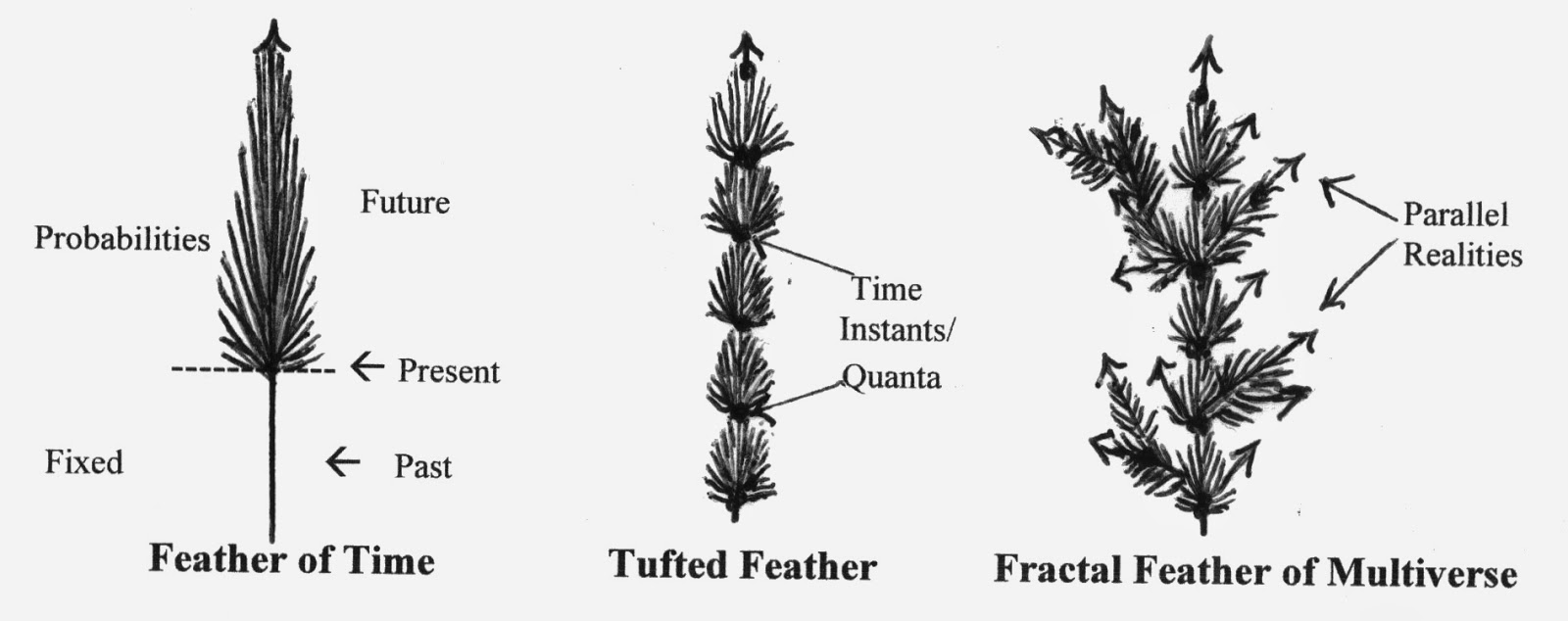FIGURE 18 - The Feather-of-Time vs. the Tufted Feather and the Fractal Feather of a Multiverse Allowing Multiple Realities and Backward Time Travel. Hawking’s feather of time is a linear time line with the present fixing the past and proceeding to one probability or another. Backward time travel not allowed (unless a new feather is created at a re-entry point in the past; in which case we would have a conditional multiverse). Tufted Feather is the same except it is broken up into a series of stacked feathers with fixed moments/time-quanta between them. Each probability tuft would collapse when a new time quantum occurred. The Fractal Feather allows all probabilities to exist as separate time lines in a Multiverse, with backward time travel conditionally allowed.

Alternative theoretical values for a time quantum, named a chronon by Robert Levi (1927), have been derived from quantum mechanics and General & Special Relativity, and were proposed to create a theory of quantum gravity. One such value proposed by Piero Caldirola (1980) is 6.97x10- 24 s, and is a value very close to that derived by Davis Mechanics. Max Planck also proposed a universal quantization of time, called Planck time, but his value was 5.39x10- 44 s. However, the one thing all such theories have in common is that they all propose a time quantum. Perhaps a universe with no quantum of time (a continuous unregulated time frame/ dimension) would come to an end or “run out” soon after it came into existence; like a clock without a regulated release of spring energy. Thus the time quantum may be a necessary and fundamental constant for regulating and maintaining the dimensions of space-time, the dimension of mass-time, and the existence of our universe.

If the speed of light is a constant because of the time quantum, then that must mean there is also a smallest meaningful unit of distance based on the unit values of c and h (perhaps the real dimensions/diameter of a black hole or an electron?); which is calculated from these constants and Davis Mechanics as 1.88x10-13 cm (Davis, 1963; Heisenberg, 1956).

REFERENCES

Campbell, John W., (1960). The Space Drive Problem. Analog Vol. LXV No. 4, June: 83-106.

Caldirola, P. (1980). "The introduction of the chronon in the electron theory and a charged lepton mass formula". Lett. Nuovo Cim. 27 (8): 225–228.

Davis, William O., (1962). The Fourth Law of Motion. Analog Vol. LXIX No. 3, May: 83-104.

Davis, William O., (1967). The Energy Transfer Delay Time. Annals of the NY Academy of Sciences, Vol. 138, 2: 862-863.

Davis, W.O., (1961) Some Unusual Implications Inherent in the Huyck Dynamic Systems Research Program. Huyck Corporation report, @1961.

Davis, W., Stine, H., Victory, E., & Korff, S., (1962). Some aspects of certain transient mechanical systems. American Phys. Soc., FA10; Spring Meeting. Wash. DC, April 23.

Dean, Norman L. (1959) US Patent # 2,886,976 - System for Converting Rotary Motion into Unidirectional Motion. US Patent Office, Wash. DC.

Encyclopaedia Britannica, Macropaedia Vol. 11, (1979). Mechanics of Complex Systems, (Principle of virtual work; Spinning tops and gyroscopes). Ency. Brit. Inc., 15th Edition. William Benton, Pub., Chicago: 777-778.

Gibbs, P (1997) . "Is The Speed of Light Constant?". In Carlip, S. Usenet Physics FAQ. University of California, Riverside. Archived from the original on 2009-11- 17. Retrieved 2009-11-26.

Gilbert, J. (1985) Harnessing g Forces. Science Digest, Sept. 1985: 14.

Harrington, Craig. http://www.inertiaHarringtonlpropulsion.com/ Inertial Propulsion Engine. A Functional Dean Drive. 12/18/2011.

Hawking, Stephen. A Brief History of Time. Bantam Books, N.Y. 1988.

Heisenberg, Werner (1958). Physics and Philosophy. Harper & Bros., NY.

Hudson, A.& Nelson, R., eds. (1982). Center of gravity/ Center of mass; The gyroscope.

University Physics. Harcourt, Brace & Jovanovich, Inc., NY: 215-219; 250-253.

Lévi, Robert (1927). "Théorie de l'action universelle et discontinue". Journal de Physique et le Radium 8 (4): 182–198. doi:10.1051/jphysrad:0192700804018200.

Margenau, Henry (1950). The Nature of Physical Reality. McGraw-Hill.

Plass, Gilbert N., (1961). Classical Electrodynamic Equations with Radiative Reaction. Review of Modern Physics 33: 37-62.

Renner, Edward (2013). “Forced Harmonic Motion in a Counter-Rotating Dual Platter System With Eccentric Rotating Sub-Platters”. Unpublished Design Prototype Paper.

Renner, Edward (1984). "A Quantum of Time: Limits, Constants, and Thermodynamics". Unpublished paper.

Stine, Harry G., (1969). Detesters, Phasers and Dean Drives. Analog Science Fiction / Science Fact Vol. No. : 61-80.

Stine, G.H., "The Rises and Falls of Henri-Marie Coanda", Air & Space Smithsonian, Sept. 1989

Von Schelling, H., (1963). Stochastic Approach to the Laws of Motion. General Electric Co., report No. 63GL106. Advanced Technology Laboratories, July 1, 1963.

Von Schelling, H., (1955) Statistische Modelle als Hilfsmittel der Naturbeschreibung. Mitteilungsblatt fuer Mathematisch Statistik 7: 173-192.

[ Ana Sayfa (Home) UFO Technology UFO's GalleriKuantum Fiziği Roket bilimi   |
[
Astronomy E-Mail   |  Index